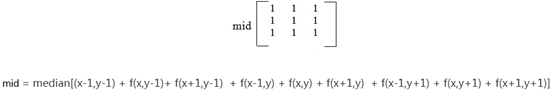# CV相关

## 仿射变换

# 2.1 创建变换矩阵
pts1 = np.float32([[50,50],[200,50],[50,200]])
pts2 = np.float32([[100,100],[200,50],[100,250]])
M = cv.getAffineTransform(pts1,pts2)
# 2.2 完成仿射变换
dst = cv.warpAffine(img,M,(cols,rows))

## 腐蚀

https://zhuanlan.zhihu.com/p/164619939

• img: 要处理的图像
• kernel: 核结构
• iterations: 腐蚀的次数，默认是1

### 闭运算

1. 礼帽运算

原图像与“开运算“的结果图之差

因为开运算带来的结果是放大了裂缝或者局部低亮度的区域，因此，从原图中减去开运算后的图，得到的效果图突出了比原图轮廓周围的区域更明亮的区域，且这一操作和选择的核的大小相关。

礼帽运算用来分离比邻近点亮一些的斑块。当一幅图像具有大幅的背景的时候，而微小物品比较有规律的情况下，可以使用顶帽运算进行背景提取。

2. 黑帽运算

为”闭运算“的结果图与原图像之差。
黑帽运算后的效果图突出了比原图轮廓周围的区域更暗的区域，且这一操作和选择的核的大小相关。
黑帽运算用来分离比邻近点暗一些的斑块。

### cv2.imshow()和plt.imshow()的区别

plt.imshow(gray,cmap=‘gray’), plt.title(‘gray’)

## 滤波

### 均值滤波cv.blur(src, ksize, anchor, borderType)

• src：输入图像
• ksize：卷积核的大小
• anchor：默认值 (-1,-1) ，表示核中心
• borderType：边界类型

### 高斯滤波

cv2.GaussianBlur(src,ksize,sigmaX,sigmay,borderType)
src: 输入图像
ksize:高斯卷积核的大小，注意 ： 卷积核的宽度和高度都应为奇数，且可以不同
sigmaX: 水平方向的标准差
sigmaY: 垂直方向的标准差，默认值为0，表示与sigmaX相同
borderType:填充边界类型

### 中值滤波cv.medianBlur(src, ksize )

# 边缘检测

## Sobel算子$$G=\sqrt{G_x^2+G_y^2}$$

sobel实现45°：

$$[[-2,-1,0], \\ [-1,0,1], \\ [0,1,2]]$$

## Canny边缘检测

1. 因为Canny容易被噪点影响，所以先进行高斯滤波器的降噪
2. 对平滑后的图像使用 Sobel 算子计算水平方向和竖直方向的一阶导数（Gx 和 Gy）。根据得到的这两幅梯度图（Gx 和 Gy）找到边界的梯度和方向1. 在每个$\theta$方向上，对边进行极大值抑制：去除非边上的点，检查所检测的像素点的周围像素，判断该检测点是否形成局部最大值
2. 滞后阈值：

现在要确定真正的边界。 我们设置两个阈值： minVal 和 maxVal。 当图像的灰度梯度高于 maxVal 时被认为是真的边界， 低于 minVal 的边界会被抛弃。如果介于两者之间的话，就要看这个点是否与某个被确定为真正的边界点相连，如果是就认为它也是边界点，如果不是就抛弃。

# 霍夫变换

### 霍夫线变换

$$\rho = x\cos\theta+y\sin\theta$$

Harris和shi-Tomas具有旋转不变性 但是不具有尺度不变性

## Harris角点检测将它求灰度值均值的公式泰勒展开后，其中有个M矩阵决定了灰度值均值的值，这个矩阵的特征向量$\lambda_1$和$\lambda_2$有如下关系：## SIFT

Harris算法可以根据它提取出的角只具有旋转不变性，但是不具有尺度不变性

### 特征点检出：

#### 提取位置：#### 提取方向### 关键点描述

ps 区域的值最终用双线性插值确定# 机器学习

## 1.性能度量

$$pre=\frac{TP}{TP+FP} \\ rec=\frac{TP}{TP+FN} \\ \frac{1}{F1}=\frac{1}{pre}+\frac{1}{rec}$$

ROC曲线：x轴假正例率，y轴真正例率，分别对应召回率和召假率

AUC曲线，基于实际的例子，按点画出的曲线，不会有ROC那么平滑，实际上一般是计算AUC的面积

## 2.线性模型

https://www.cnblogs.com/muzixi/p/6642203.html

$$f(x)=wx+b \\ (w^*,b^*)=\begin{array} aargmin \\ (w,b) \end{array} \ \sum^m_{i=1}(f(x_i)-y_i)^2$$

## 3.感知模型

### 感知机（线性模型加了判断）：

$$f(x)=sign(wx+b)\\ sign(x) \begin{cases} +1 \ , \ x\ge0 \\ -1 \ , \ x<0 \end{cases}$$

（误分类说明预测的分类 (wx+b)和实际分类不一致，因此乘积为-1，而距离是绝对值，所以应该是 -y(wx+b)））

$$L(w^*,b^*)=\begin{array} aargmin \\ (w,b) \end{array} \ -\sum^m_{i=1}y_if(x_i)$$

ps 和SVM的区别：## 4.逻辑回归

$$h_\theta(x)=g(\theta^Tx)=\frac{1}{1+e^{-\theta x}}$$

$$J(\theta)=\sum^m_{i=1}[y_i log h_\theta(x_i)+(1-y_i)log(1-h_\theta(x_i))]$$

## 6.决策树

https://blog.csdn.net/gunhunti4524/article/details/81506012

### ID3：

ID3 仅仅适用于二分类问题。ID3 仅仅能够处理离散属性。

$$Ent(D)=-\sum^{|y|}_{k=1}p_klog_2p_k$$

$$Gain(D,a)=Ent(D)-\sum^V_{v=1}\frac{|D^v|}{|D|}Ent(D^v)$$

（例如a=颜色时，v_1=绿色，v_2=黄色）

### C4.5:

C4.5 使用信息增益比作为选择特征的准则；

$$Gain\_ratio(D,a)=\frac{Gain(D,a)}{H(a)} \\ H(a)=-\sum^V_{v=1}\frac{|D^v|}{|D|}\log_2\frac{|D^v|}{|D|}$$

### CART

CART 使用 Gini 指数作为选择特征的准则。

CART 的全称是分类与回归树（classification and regression tree）。从这个名字中就应该知道，CART 既可以用于分类问题，也可以用于回归问题。

$$Gini(D)=\sum^{|y|}_{k=1}\sum_{k\neq1}p_kp_{k}=1-\sum^{|y|}_{k=1}p^2_k$$

$$Gini\_index(D,a)=\sum^V_{v=1}\frac{|D^v|}{|D|}Gini(D^v)$$

Gini 指数 vs 熵

Gini 指数的计算不需要对数运算，更加高效；

Gini 指数更偏向于连续属性，熵更偏向于离散属性。

## 7.贝叶斯

$$P(c|x)=\frac{P(x,c)}{P(x)}=\frac{P(x|c)P(c)}{P(x)}$$

$$P(c)=\frac{|D_c|}{|D|}$$

D为数据集

### 极大似然估计

$$P(D_c|\theta_c)=\prod_{x\in D_c}P(x|\theta_c)$$

$$LL(\theta_c)=\log \,P(D_c|\theta_c) \\ = \sum_{x\in D_c} \, \log P(x|\theta_c)$$

$$\hat \theta_c =\ \underset {\theta_c}{arg \, max}LL(\theta_c)$$

### 朴素贝叶斯

$$P(c|x)=\frac{P(x|c)P(c)}{P(x)}=\frac{P(c)}{P(x)}\prod^d_{i=1} P(x_i|c)$$

d为属性数目，$x_i$为x在第i个属性上的取值

$$h_{nb}(x) = \underset {c\in y} {arg \, max} P(c)\prod^d_{i=1} P(x_i|c)$$

$$P(x_i|c)=\frac{|D_{c,x_i}|}{|D_c|}$$